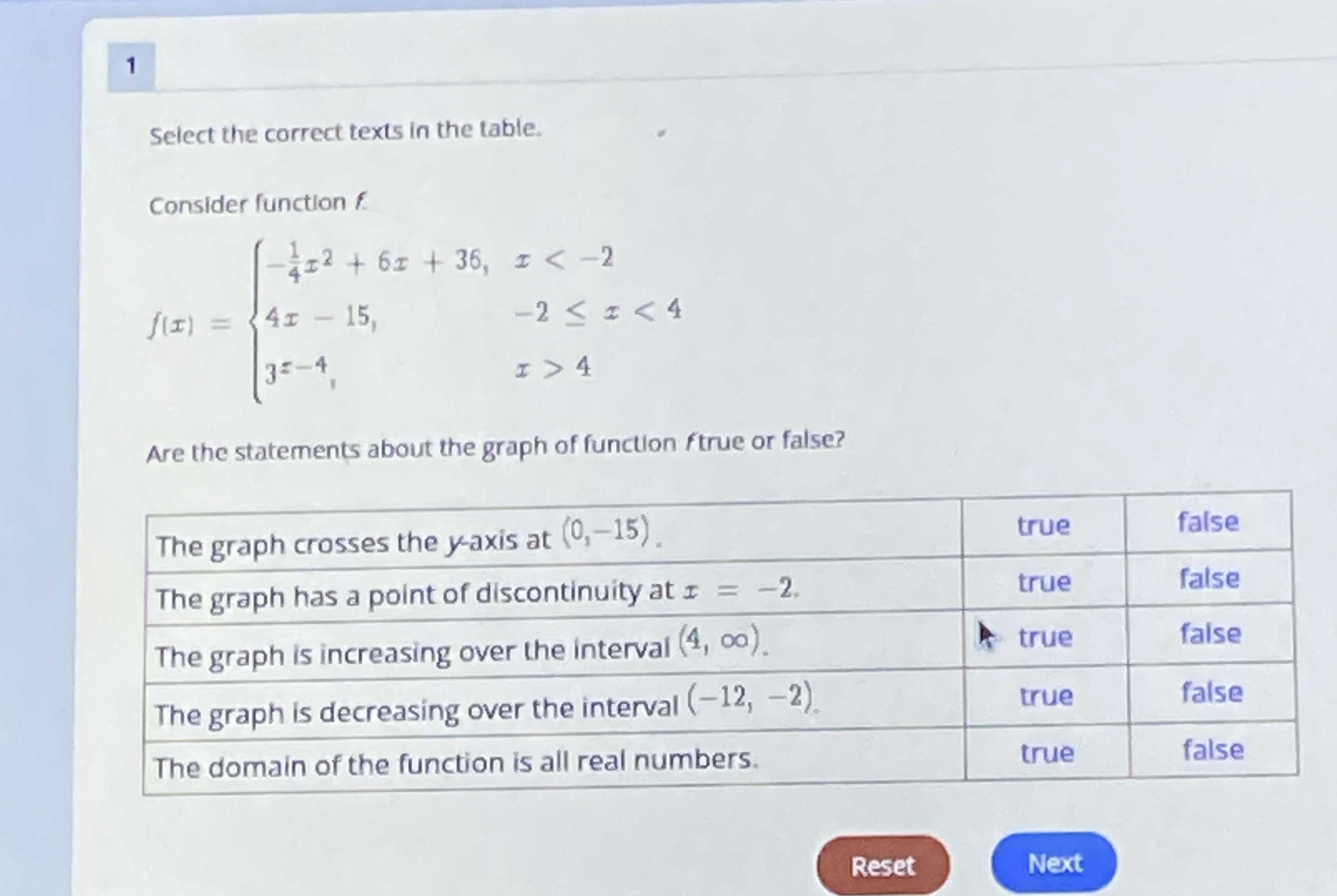### Still have math questions?

Algebra
QuestionSelect the correct texts in the table. Consider function $$f$$

$$f ( x ) = \left\{ \begin{array} { l l } { - \frac { 1 } { 4 } x ^ { 2 } + 6 x + 36 , } & { x < - 2 } \\ { 4 x - 15 , } & { - 2 \leq x < 4 } \\ { 3 z - 4 , } & { x > 4 } \end{array} \right.$$

Are the statements about the graph of function ftrue or false?

The graph crosses the $$y$$ -axis at $$( 0 , - 15 )$$

The graph has a point of discontinuity at $$x = - 2$$ .

The graph is increasing over the interval $$( 4 , \infty )$$ .

The graph is decreasing over the interval $$( - 12 , - 2 )$$

The domain of the function is all real numbers.